Note: this document will print in an appropriately modified format 7 pages)

1  Dissociation of water

The ability of acids to react with bases depends on the tendency of hydrogen ions to combine with hydroxide ions to form water:

H+(aq) + OH(aq) → H2O(1-1)

This tendency happens to be very great, so the reaction is practically complete— but not "completely" complete; a few stray H+ and OH ions will always be present. What's more, this is true even if you start with the purest water attainable. This means that in pure water, the reverse reaction, the "dissociation" of water

H2O → H+(aq) + OH(aq) (1-2)

will proceed to a very slight extent. Both reactions take place simultaneously, but (1) is so much faster than (2) that only a minute fraction of H2O molecules are dissociated.

Liquids that contain ions are able to conduct an electric current. Pure water is practically an insulator, but careful experiments show that even the most highly purified water exhibits a very slight conductivity that corresponds to a concentration of both the H+ ion and OH ions of almost exactly 1.00 × 10–7mol L–1 at 25°C.

The ion product of water

The degree of dissociation of water is so small that you might wonder why it is even mentioned here. The reason stems from an important relationship that governs the concentrations of H+ and OH ions in aqueous solutions:

[H+][OH] = 1.00 × 10–14Kw(1-3)Must know!

The quantity 1.00 x 10–14 is commonly denoted by Kw. Its value varies slightly with temperature, pressure, and the presence of other ions in the solution.

in which the square brackets [ ] refer to the concentrations (in moles per litre) of the substances they enclose.

This expression is known as the ion product of water, and it applies to all aqueous solutions, not just to pure water. The consequences of this are far-reaching, because it implies that if the concentration of H+ is large, that of OH will be small, and vice versa. This means that H+ ions are present in all aqueous solutions, not just acidic ones.

This leads to the following important definitions, which you must know:

 acidic solution [H+] > [OH–] alkaline ("basic") solution [H+] < [OH–] neutral solution [H+] = [OH–] = 1.00×10–7 mol L–1

Take special note of the followng definition:

A neutral solution is one in which the concentrations of H+ and OH ions are identical.

The values of these concentrations are constrained by (1-3). Thus, in a neutral solution, both the hydrogen- and hydroxide ion concentrations are 1.00 × 10–7 mol L–1:

[H+][OH] = [1.00 × 10–7][1.00 × 10–7] =1.00 × 10–14

A typical strong acid

Hydrogen chloride is a gas that readily dissolves in water to form an acidic solution which we call hydrochloric acid (whose formula is also HCl.) Recall that strong acids are totally dissociated in solution, as was indicated in (1-2) near the beginning of this section:

HCl → H+(aq) + Cl(aq)

Because this dissociation is essentially complete, a 1.0M solution of HCl in water does not really contain any significant concentration of HCl molecules at all; it is a solution of H+ and Cl in which the concentrations of both ions are 1.0 mol L–1. The concentration of hydroxide ion in such a solution, according to (1-3), is

[OH] = (Kw)/[H+] = (1.00 x 10–14) / (1 mol L–1) = 1.00 x 10–14 mol L–1.

Similarly, the concentration of hydrogen ion in a solution made by dissolving 1.0 mol of sodium hydroxide in water will also be 1.00 x 10–14 mol L–1.

2  Understanding pH

When dealing with a range of values (such as the hydrogen ion concentrations encountered in chemistry) that spans many powers of ten, it is convenient to represent them on a more compressed logarithmic scale. By convention, we use the pH scale to denote hydrogen ion concentrations:

Eqn 1b follows from 1a. If this fact is not apparent to you, please consider brushing up on logarithms; it will save you a lot of trouble in the long run!

pH = – log10 [H+](2-1a)must know!

or conversely,

[H+] = 10–pH(2-1b)This notation was devised by the Danish chemist Søren Sørensen (1868-1939) in 1909. There are several accounts of why he chose "pH"; a likely one is that the letters stand for the French term pouvoir hydrogène, meaning "power of hydrogen"— "power" in the sense of an exponent. It has since become common to represent other small quantities in "p-notation". Two that you need to know in this course are the following:

pOH = – log10 [OH]

pKw = – log Kw (= 14 when Kw = 1.00 × 10–14)

Note that pH and pOH are expressed as numbers without any units, since logarithms must be dimensionless.

Recall equation (1-3) for the ion product of water:

[H+][OH] = 1.00 × 10–14

if we write this in "p-notation" it becomes

pH + pOH = 14(2-2)must know!

In a neutral solution at 25°C, pH = pOH = 7.0. As pH increases, pOH diminishes; a pH greater than 7.0 corresponds to an alkaline solution, a pH of less than 7.0 is an acidic solution. In a solution with [H+] = 1 M , the pH would be 0; in a 0.00010 M solution of H+, it would be 4.0. Similarly, a 0.00010 M solution of NaOH would have a pOH of 4.0, and thus a pH of 10.0. It is very important that you thoroughly understand the pH scale, and be able to convert between [H+] or [OH] and pH in both directions. Must know!

The pH scale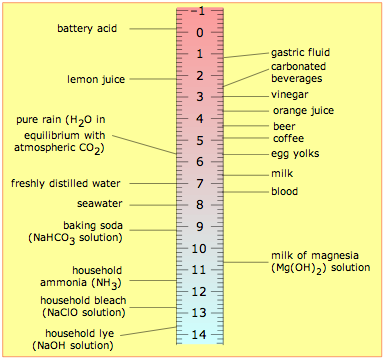The range of possible pH values runs from about 0 to 14.

The word "about" in the above statement reflects the fact that at very high concentrations (10 M hydrochloric acid or sodium hydroxide, for example,) a significant fraction of the ions will be associated into neutral pairs such as H+·Cl, thus reducing the concentration of “available” ions to a smaller value which we will call the effective concentration. It is the effective concentration of H+ and OH that determines the pH and pOH. For solutions in which ion concentrations don't exceed 0.1 M, the formulas pH = –log [H+] and pOH = –log[OH] are generally reliable, but don't expect a 10.0 M solution of a strong acid to have a pH of exactly –1.00!

The image above will help give you a general feeling for where common substances fall on the pH scale. Notice especially that

• most foods are slightly acidic;
• the principal "bodily fluids" are slightly alkaline, as is seawater— not surprising, since early animal life began in the oceans.
• the pH of freshly-distilled water will drift downward as it takes up carbon dioxide from the air; CO2 reacts with water to produce carbonic acid, H2CO3.
•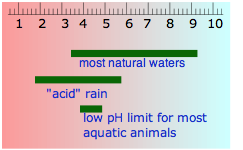the pH of water that occurs in nature varies over a wide range. Groundwaters often pick up additional CO2 respired by organisms in the soil, but can also become alkaline if they are in contact with carbonate-containing sediments. "Acid" rain is by definition more acidic than pure water in equilibrium with atmospheric CO2, owing mainly to sulfuric and nitric acids that originate from fossil-fuel emissions of nitrogen oxides and SO2.

pH indicators

The colors of many dye-like compounds depend on the pH, and can serve as useful indicators to determine whether the pH of a solution is above or below a certain value.

Here is a list of ordinary foods and household substances that can serve as indicators

Natural indicator dyes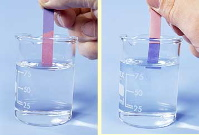The best known of these is of course litmus, which has served as a means of distinguishing beween acidic and alkaline substances since the early 18th century.Many flower pigments are also dependent on the pH. You may have noticed that the flowers of hydrangea shrubs are blue when grown in acidic soils, and white or pink in alkaline soils.

Red cabbage is a popular make-it-yourself indicator. Here is a typical recipe.

Universal indicators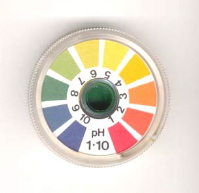Most indicator dyes show only one color change, and thus are only able to determine whether the pH of a solution is greater or less than the value that is characteristic of a particular indicator. By combining a variety of dyes whose color changes occur at different pHs, a "universal" indicator can be made. Commercially-prepared pH test papers of this kind are available for both wide and narrow pH ranges.

3  Acid-base titration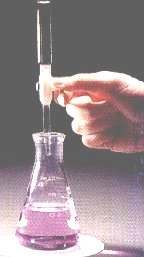Since acids and bases readily react with each other, it is experimentally quite easy to find the amount of acid in a solution by determining how many moles of base are required to neutralize it. This operation is called titration, and you should already be familiar with it from your work in the Laboratory.

We can titrate an acid with a base, or a base with an acid. The substance whose concentration we are determining (the analyte) is the substance being titrated; the substance we are adding in measured amounts is the titrant. The idea is to add titrant until the titrant has reacted with all of the analyte; at this point, the number of moles of titrant added tells us the concentration of base (or acid) in the solution being titrated.

Interpreting titraion curves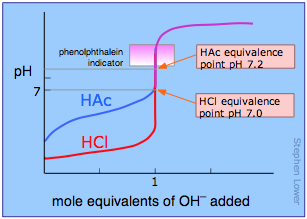The course of a titration can be followed by plotting the pH of the solution as a function of the quantity of titrant added. The figure shows two such curves, one for a strong acid (HCl) and the other for a weak acid, acetic acid, denoted by HAc. Looking first at the HCl curve, notice how the pH changes very slightly until the acid is almost neutralized. At that point, which corresponds to the vertical part of the plot, just one additional drop of NaOH solution will cause the pH to jump to a very high value— almost as high as that of the pure NaOH solution.

Compare the curve for HCl with that of HAc. For a weak acid, the pH jump near the neutralization point is less steep. Notice also that the pH of the solution at the neutralization point is greater than 7. These two characteristics of the titration curve for a weak acid are very important for you to know.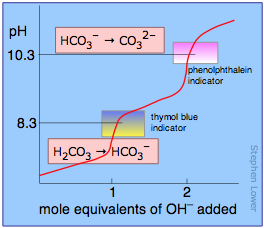If the acid or base is polyprotic, there will be a jump in pH for each proton that is titrated. In the example shown here, a solution of carbonic acid H2CO3 is titrated with sodium hydroxide. The first equivalence point (at which the H2CO3 has been converted entirely into bicarbonate ion HCO3) occurs at pH 8.3. The solution is now identical to one prepared by dissolving an identical amount of sodium bicarbonate in water.

Addition of another mole equivalent of hydroxide ion converts the bicarbonate into carbonate ion and is complete at pH 10.3; an identical solution could be prepared by dissolving the appropriate amount of sodium carbonate in water.

Finding the equivalence point: indicators

When enough base has been added to react completely with the hydrogens of a monoprotic acid, the equivalence point has been reached. If a strong acid and strong base are titrated, the pH of the solution will be 7.0 at the equivalence point. However, if the acid is a weak one, the pH will be greater than 7; the “neutralized” solution will not be “neutral” in terms of pH. For a polyprotic acid, there will be an equivalence point for each titratable hydrogen in the acid. These typically occur at pH values that are 4-5 units apart, but they are occasionally closer, in which case they may not be readily apparent in the titration curve.

The key to a successful titration is knowing when the equivalance point has been reached. The easiest way of finding the equivalence point is to use an indicator dye; this is a substance whose color is sensitive to the pH. One such indicator that is commonly encountered in the laboratory is phenolphthalein; it is colorless in acidic solution, but turns intensely red when the solution becomes alkaline. If an acid is to be titrated, you add a few drops of phenolphthalein to the solution before beginning the titration. As the titrant is added, a local red color appears, but quickly dissipates as the solution is shaken or stirred. Gradually, as the equivalence point is approached, the color dissipates more slowly; the trick is to stop the addition of base after a single drop results in a permanently pink solution.

Different indicators change color at different pH values; see here for an illustrated list. Since the pH of the equivalance point varies with the strength of the acid being titrated, one tries to fit the indicator to the particular acid. One can titrate polyprotic acids by using a suitable combination of several indicators, as is illustrated above for carbonic acid.

Concept Map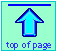Chem1 pH and Titrationintroduces thls subjecc for a course in General Chemistry. It is part of the General Chemistry Virtual Textbook, a free, online reference textbook for General Chemistry by of Simon Fraser University .

This chapter covers the following topics at an elementary level: ion product of water, understanding pH, acid-base titration. . It can be accessed directly at .